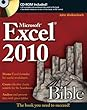## Excel Book Recommendation

I am often asked to recommend an Excel book. This isn’t always easy, as different people need different things from a book, depending not only on their Excel ability and aspirations, but also their personality and learning preferences.One consistent recommendation I do give, though, is for John Walkenbach’s Excel Bibles:
Excel 2010 Bible – John Walkenbach – Amazon UKIf you only want to get ONE Excel book, this would be it. There’s a bible for each version of Excel, and they’re an excellent mix of how-to guides, background information and reference material.

## Index / Match Formula – Basics

INDEX and MATCH are two of the most powerful and flexible functions in Excel – but they are also two of the least understood. I often meet users who are confident with VLOOKUP and HLOOKUP formulae, but don’t seem to understand INDEX and MATCH. So I thought I’d provide a basic guide to using these functions.

### INDEX

This simply returns a value from a list, based on its position in the list.

The INDEX function requires 2 arguments; The first is the list of values, or range containing the list of values. The second argument is the position in that list from which to return a value.Example:  Who is the 4th name in this list? We can use INDEX to tell us:

=INDEX(A2:A9,4)

Which returns “Roger”.

### MATCH

Now, the MATCH function does the exact opposite of INDEX: it returns the position in a list where a specific value occurs.

The MATCH function requires 3 arguments; The first is the value we want to find, the second is the list (or range containing the list) that we want to look in, and the third argument specifies whether we want an exact match, or the next lowest / highest.Example: Which position is Helen in, in our example data:

=MATCH(“Helen”,A2:A9,0)

This formula returns the answer 3.

Note the third argument – 0 – which tells Excel we want an EXACT match.

### INDEX / MATCH as a LOOKUP

These functions are each useful on their own. But when we combine them, they become even more powerful. Let’s look at the example data again, and ask the question:
Who drives the Hyundai?Now, if the columns were reversed, you could simply use a VLOOKUP, to return the Name based on the Car value. So, you could restructure your data. Or you could add a helper column, to repeat the name in column C, and then use a VLOOKUP.

But it’s not always possible or practical to restructure a workbook, and it’s certainly not efficient to duplicate data. What we really want is a “left-looking” VLOOKUP – and this is where INDEX / MATCH can be used so effectively.

We can use MATCH to return the position in the list of Hyundai:
=MATCH(“Hyundai”,B2:B9,0)
This returns 6.

Now we can use the INDEX function to find the sixth name in the list:
=INDEX(A2:A9,6)
Which tells us it’s Ann.

Now we can simply combine the functions in one formula:
=INDEX(A2:A9,MATCH(“Hyundai”,B2:B9,0))
And with one little formula, we get the answer we wanted!

There are more advanced capabilities of both INDEX and MATCH functions, including 2 dimensional arrays, multiple areas, and returning closest values. But this post covers the basic use of the INDEX and MATCH functions in Excel.Download Example File